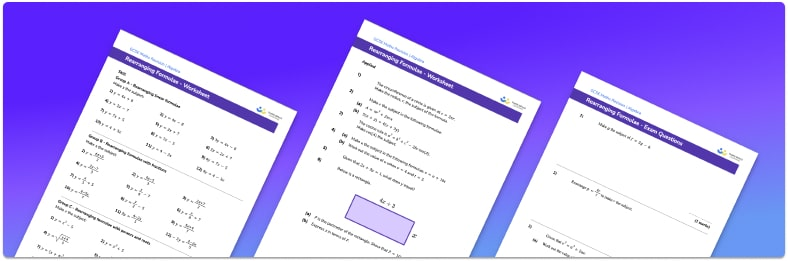# Rearranging Formulae Worksheet• Section 1 of the rearranging formulae worksheet contains 20+ skills-based rearranging formulae questions, in 3 groups to support differentiation
• Section 2 contains 3 applied rearranging formulae questions with a mix of worded problems and deeper problem solving questions
• Section 3 contains 3 foundation and higher level GCSE exam questions on rearranging formulae
• Answers and a mark scheme for all rearranging formulae questions are provided
• Questions follow variation theory with plenty of opportunities for students to work independently at their own level
• All questions created by fully qualified expert secondary maths teachers
• Suitable for GCSE maths revision for AQA, OCR and Edexcel exam boards

• This field is for validation purposes and should be left unchanged.

You can unsubscribe at any time (each email we send will contain an easy way to unsubscribe). To find out more about how we use your data, see our privacy policy.

### Rearranging formulae at a glance

We can rearrange a formula, or change the subject of the formula, so that one unknown is alone on the left-hand side of the formula and is equal to the rest of the formula. This allows us to work out the value of that unknown, when we know the other values. We rearrange formulae by applying inverse functions.

For example, if we have the formula for the circumference of a circle, C=π times d, we can rearrange the formula to make d the subject, which will help us to work out the diameter, given the circumference of a circle. At the moment, d is multiplied by π. To move π to the other side of the equation, we apply the inverse of this, which is divide by π. If we divide both sides by π we get C divided by π = d which can be written as d = C divided by π. We have now made π the subject of the formula.

It is important to remember that the inverse function to the square of a number is a square root. The square root of a number can be written as the number, raised to the power of a half. The square, and the square root of a number are both powers (indices). This is also true for the cube, and the inverse, the cube root.

Common equations used to test knowledge of rearranging formulae are: area of 2D shapes, surface area / volume of 3D shapes, converting units such as celsius to fahrenheit, and equations of a straight line.

Looking forward, students can then progress to additional rearranging equations worksheets and other algebra worksheets, for example a sequences worksheet, simultaneous equations worksheet or straight line graphs worksheet.For more teaching and learning support on Algebra our GCSE maths lessons provide step by step support for all GCSE maths concepts.

## Do you have KS4 students who need more focused attention to succeed at GCSE?There will be students in your class who require individual attention to help them succeed in their maths GCSEs. In a class of 30, it’s not always easy to provide.

Help your students feel confident with exam-style questions and the strategies they’ll need to answer them correctly with our dedicated GCSE maths revision programme.

Lessons are selected to provide support where each student needs it most, and specially-trained GCSE maths tutors adapt the pitch and pace of each lesson. This ensures a personalised revision programme that raises grades and boosts confidence.

Find out more